# Precalculus : Products and Quotients of Complex Numbers in Polar Form

## Example Questions

1 3 Next →

### Example Question #13 : Find The Quotient Of Complex Numbers

Divide.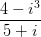Possible Answers: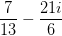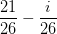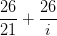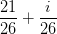Correct answer:Explanation:

To divide complex numbers, multiply both the numerator and denominator by the conjugate of the denominator.

To find the conjugate, just change the sign in the denominator. The conjugate used will be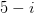.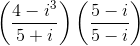Now, distribute and simplify.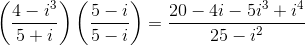Recall that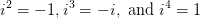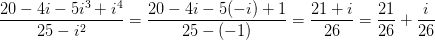### Example Question #21 : Products And Quotients Of Complex Numbers In Polar Form

Divide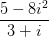Possible Answers: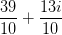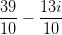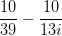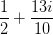Correct answer:Explanation:

Start by simplifying the fraction.

Recall that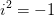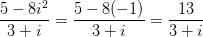To divide complex numbers, multiply both the numerator and denominator by the conjugate of the denominator.

To find the conjugate, just change the sign in the denominator. The conjugate used will be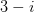.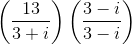Now, distribute and simplify.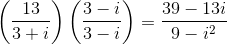Recall that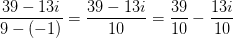### Example Question #15 : Find The Quotient Of Complex Numbers

Simplify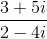into a number of the form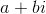.

Possible Answers: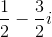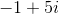None of the other answers.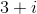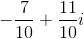Correct answer:Explanation:

We haveMultiply by the complex conjugate of the denominator.

The complex conjugate is the denominator with the sign changed: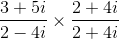Multiply fractions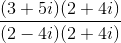FOIL the numerator and denominator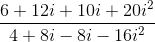Apply the rule of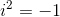: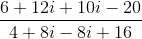Simplify.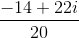Simplify further using the addition of fractions rule, then factor the i out of the 2nd fraction.### Example Question #16 : Find The Quotient Of Complex Numbers

Divide: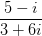Possible Answers: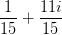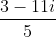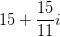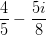Correct answer:Explanation:

To divide complex numbers, multiply both the numerator and denominator by the conjugate of the denominator.

To find the conjugate, just change the sign in the denominator. The conjugate used will be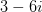.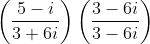Now, distribute and simplify.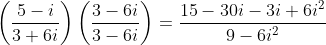Recall that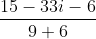Then combine like terms: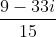Then since each term is a multiple of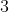you can simplify:1 3 Next →

### All Precalculus Resources